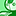# QlikView App Dev

Discussion Board for collaboration related to QlikView App Development.

Announcements
Our next Qlik Insider session will cover new key capabilities. Join us August 11th REGISTER TODAY
cancel
Showing results for
Did you mean:Partner

## help combining variables

Hi all,

i have the following variables..

vCounter = Only({<nd_FISCAL_PERIOD = {\$(=Only(CurrentFiscalPeriod))}>}Counter)

vPeriod_1 = Only({<Counter = {\$(=vCounter-1)}>} nd_FISCAL_PERIOD)

as you can see, vPeriod_1 uses vCounter within it.

i need to combine the above 2 variables, the vCounter expression needs to replace the vCounter part of the vPeriod_1 variable expression.

then the i want to replace the vPeriod_1 part of the below expression with the combined expression from the above.

='("LV Snapshot FY\$(=Right(CurrentFiscalYear,2))*" & (*\$(vPeriod_1)))'

i cant get this to work, can anyone help please?

i have attached an example qvw.

thanks

15 RepliesMVP

Sorry, I don't know NPrinting good enough.

Qlik NPrintingPartner
Author

last question i promise!

i now need to add in the additional 2 periods (201703 and 201702) which are from the other 2 variables you did.

for this to work in NP i would need the result to be comma separated, (i think) so the 3 values can be selected the same time.

is it possible to add these to the following expression?

='LV Snapshot FY\$(=Right(CurrentFiscalYear,2)) \$(=Only({<Counter = {\$(=Only({<nd_FISCAL_PERIOD = {\$(=Only(CurrentFiscalPeriod))}>}Counter)-1)}>} nd_FISCAL_PERIOD))'MVP

Maybe like

='LV Snapshot FY\$(=Right(CurrentFiscalYear,2)) \$(=Only({<Counter = {\$(=Only({<nd_FISCAL_PERIOD = {\$(=Only(CurrentFiscalPeriod))}>}Counter)-1)}>} nd_FISCAL_PERIOD)),LV Snapshot FY\$(=Right(CurrentFiscalYear,2)) \$(=Only({<Counter = {\$(=Only({<nd_FISCAL_PERIOD = {\$(=Only(CurrentFiscalPeriod))}>}Counter)-2)}>} nd_FISCAL_PERIOD)),LV Snapshot FY\$(=Right(CurrentFiscalYear,2)) \$(=Only({<Counter = {\$(=Only({<nd_FISCAL_PERIOD = {\$(=Only(CurrentFiscalPeriod))}>}Counter)-3)}>} nd_FISCAL_PERIOD))'Partner
Author

that works in a text box, but how would i set it up in an action on the button to make the 3 selections on the Dataset field?MVP

Don't know how NPrinting works, but in QV, you can use a compound search like

("value1"|"value2"|"value3")Partner
Author

thanks for all your helpi have changed the expression to the following..

='("LV Snapshot FY\$(=Right(CurrentFiscalYear,2)) \$(=Only({<Counter = {\$(=Only({<nd_FISCAL_PERIOD = {\$(=Only(CurrentFiscalPeriod))}>}Counter)-1)}>} nd_FISCAL_PERIOD))"|"LV Snapshot FY\$(=Right(CurrentFiscalYear,2)) \$(=Only({<Counter = {\$(=Only({<nd_FISCAL_PERIOD = {\$(=Only(CurrentFiscalPeriod))}>}Counter)-2)}>} nd_FISCAL_PERIOD))"|"LV Snapshot FY\$(=Right(CurrentFiscalYear,2)) \$(=Only({<Counter = {\$(=Only({<nd_FISCAL_PERIOD = {\$(=Only(CurrentFiscalPeriod))}>}Counter)-3)}>} nd_FISCAL_PERIOD))")'

which works in QV but i can't get it to work in NP.

i have asked Stephen Jasonowski at Qlik for support.

thanks again!Community Browser